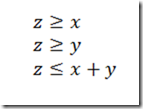## Thursday, May 3, 2012

### Binary OR

Is it possible to implement a kind of a boolean addition to binary variables.

For example:

Let X, Y and Z be binary variables.

X and Y ara inputs, Z is a result.

X + Y = Z

Such that

0 + 0 = 0

0 + 1 = 1

1 + 0 = 1

1 + 1 = 1

So this would be sometning like a logical .OR. addition?

This can be formulated as a set of inequalities:The variable Z can be relaxed to a continuous variable between 0 and 1 (it will be integer automatically).

1.2.